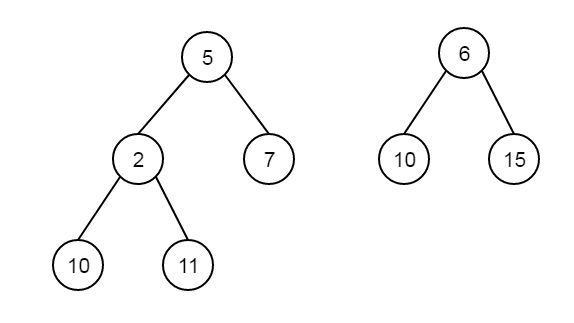# Find first non matching leaves in two binary trees in C++

Suppose we have two binary trees. We have to find first leaf of two trees, that does not match. If there are no non-matching leaves, then display nothing.If these are two trees, then the first non-matching leaves are 11 and 15.

Here we will use the iterative preorder traversal of both of the trees simultaneously using stack. We will use different stack for different trees. We will push nodes into the stack till the top node is the leaf node. Compare two top, if they are same, then do further checking, otherwise show two stack top elements.

## Example

Live Demo

#include <iostream>
#include <stack>
using namespace std;
class Node {
public:
int data;
Node *left, *right;
};
Node *getNode(int x) {
Node * newNode = new Node;
newNode->data = x;
newNode->left = newNode->right = NULL;
return newNode;
}
bool isLeaf(Node * t) {
return ((t->left == NULL) && (t->right == NULL));
}
void findUnmatchedNodes(Node *t1, Node *t2) {
if (t1 == NULL || t2 == NULL)
return;
stack<Node*> s1, s2;
s1.push(t1); s2.push(t2);
while (!s1.empty() || !s2.empty()) {
if (s1.empty() || s2.empty() )
return;
Node *top1 = s1.top();
s1.pop();
while (top1 && !isLeaf(top1)){
s1.push(top1->right);
s1.push(top1->left);
top1 = s1.top();
s1.pop();
}
Node * top2 = s2.top();
s2.pop();
while (top2 && !isLeaf(top2)){
s2.push(top2->right);
s2.push(top2->left);
top2 = s2.top();
s2.pop();
}
if (top1 != NULL && top2 != NULL ){
if (top1->data != top2->data ){
cout << "First non matching leaves are: "<< top1->data <<" "<< top2->data<< endl;
return;
}
}
}
}
int main() {
Node *t1 = getNode(5);
t1->left = getNode(2);
t1->right = getNode(7);
t1->left->left = getNode(10);
t1->left->right = getNode(11);
Node * t2 = getNode(6);
t2->left = getNode(10);
t2->right = getNode(15);
findUnmatchedNodes(t1,t2);
}

## Output

First non matching leaves are: 11 15